Disclosure: This post may contain affiliate links, meaning when you click the links and make a purchase, we receive a commission.

# How to insert Equation in Excel using Equation Editor (Easy Guide)

[Abstract: In this article, you will learn how to insert equation in Excel using Equation Editor with two examples.]

If you do mathematics related report or assignment with Excel, you have to know how to use the Equation Editor.

In this tutorial, we shall learn how to use the Equation Editor Effectively.

Click on the Insert Tab. In the “Symbols” group of commands, you will find a split button: it is Equation.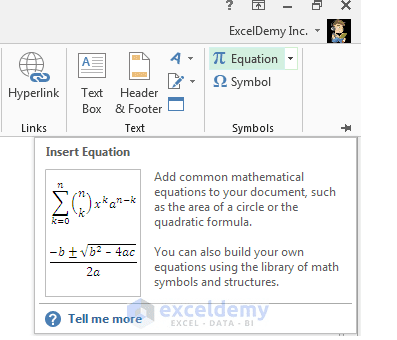Equation command in the Symbols group of commands in the Insert tab.

To insert an Equation Editor, just click on the button, an equation Editor appears.Excel Equation Editor

You can also insert predefined equations clicking on the drop down part of the Equation command. You see a list of pre-built equations are showing in the drop down list.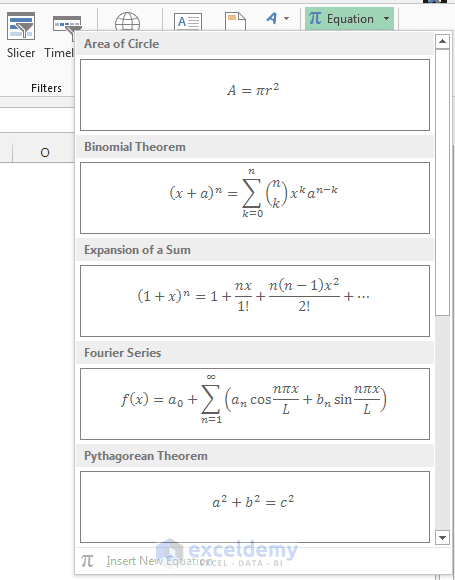Pre-built equations

I insert the Binomial Theorem Equation from the list.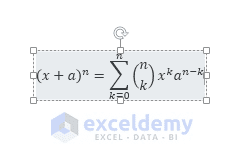Binomial Theorem Equation

OK.

I don’t need the binomial equation right now.

Want to see the whole process with a video lecture?

Join my course at Udemy (100% Off)!

Excel Charts & Graphs and SmartArt Graphics for Beginners

## Step by step process to insert an equation using Equation Editor

I am going to create two sample equations step by step to show you the whole process of using an equation editor.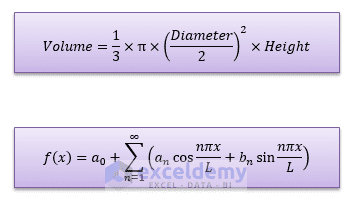I shall show how to insert these two equations in Excel using Equation Editor.

### Inserting First Equation

At first I shall create the Volume formula. I click on the Insert tab and then click on the Equation command, the Equation Editor appears.Excel Equation Editor

When Equation Editor is selected, two contextual tabs appear in the tab list. One is the Drawing Tools Format contextual tab. Equation Editor is a shape. You can format the shape using the commands under the Format contextual tab of Drawing Tools.

The other tab is Equation Tools Design contextual tab. It will help you to insert an equation into the Equation Editor.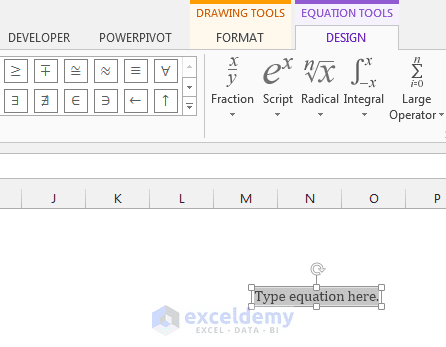Drawing Tools Format and Equation Tools Design contextual tabs

The first command in the Equation Tools Design contextual tab is the Equation command. The built in equations are listed in this drop down list.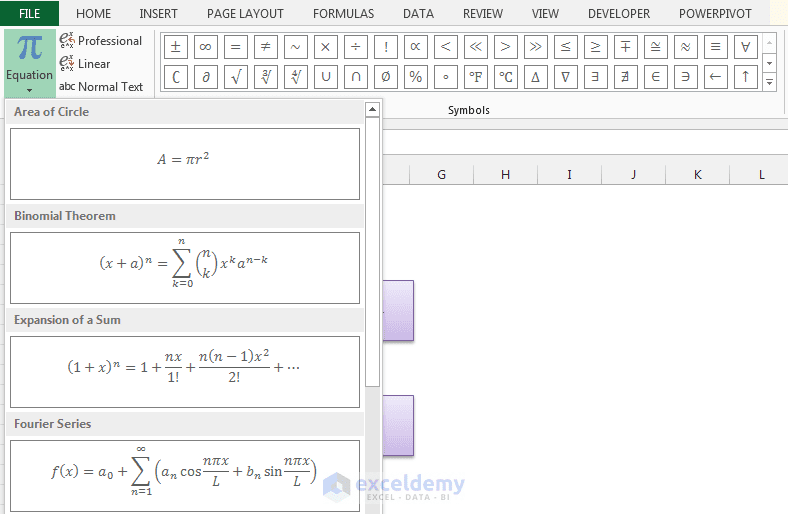Equation drop down in the Design contextual tab.

Then there are some symbols. You can use these symbols in the equation. To see more symbols click on the More button [on the bottom right corner of the Symbols window].

The window is expanded, in the window there is a drop down on the top right corner. Right now “Basic Math” symbols are showing in the window. Click on the drop down.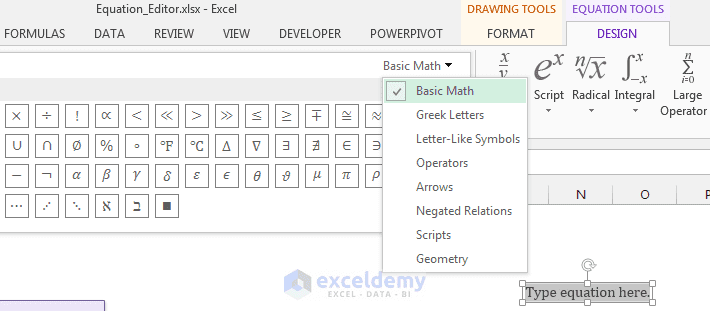Except Basic Math, you can also work with these symbol categories.

You can work with several options:

1. Basic Math
2. Greek Letters
3. Letter-Like Symbols
4. Operators
5. Arrows
6. Negated Relations
7. Scripts
8. Geometry

If you select Greek Letters, you will  get two types of Greek Letters: Lower case Greek letters, and upper case Greek Letters.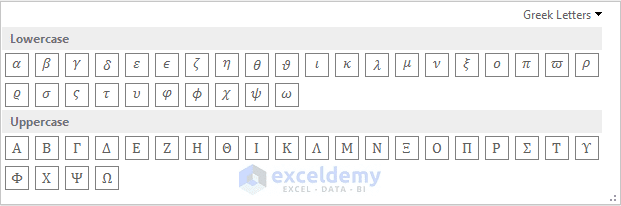Greek Letters

If you select Letter-Like Symbols, you will get symbols like regular letters.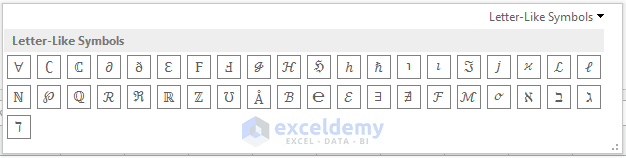Letter Like symbols

If you select Operators category, you will get a good number of sub-categories in it. They are: Common Binary Operators, Common Relational Operators, Basic N-ary operators, then Advanced Binary Operators and Advanced Relational Operators.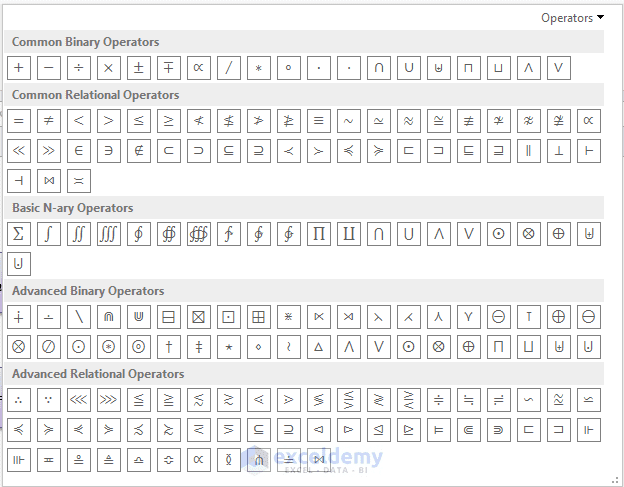Operators

If you select Arrows, you will get different types of arrow symbols.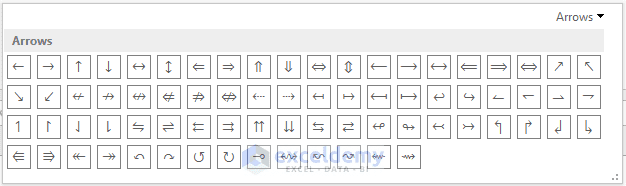Arrows

Under Negated Relations you will get the symbols like the following image.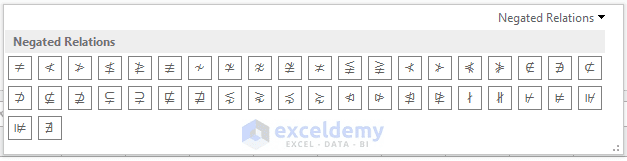Negated Relations Symbols

Then Scripts, so many Script categories…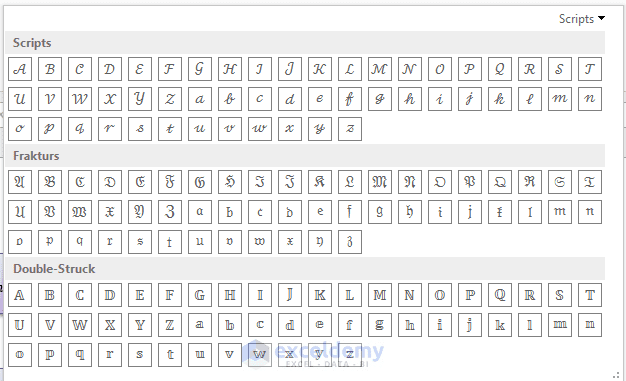Scripts

and finally you can work with the symbols of Geometry.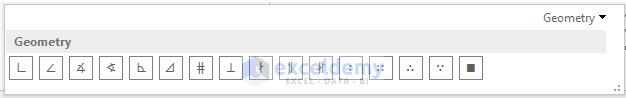Geometry symbols

There are some Structures on the right side of the Symbols group of commands.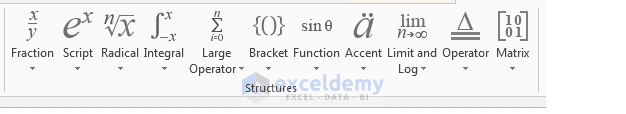Structures

At first you will find Fraction type structure. In the blank boxes you can input values, just the structure will be like the following image.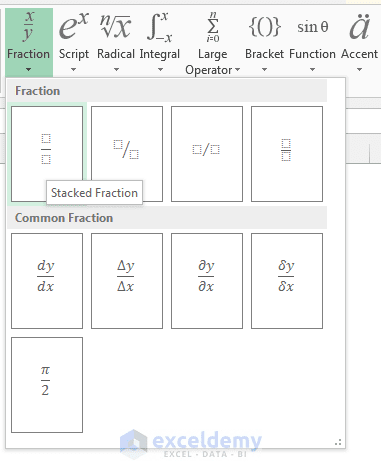Fraction Structures

Then Script type structure, then Radical, Integral, Large Operator, Bracket, Trigonometric Functions, Inverse Functions, Accent, Limit and Logarithm, Operator and finally the Matrix structure.

Now I type in the equation editor Volume, then equal sign, you can see from the volume equation that it has a fraction, so I click on the Fraction drop down from the Structures group of commands and I select the Stacked Fraction.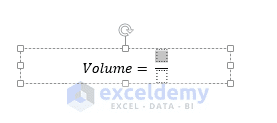Stacked Fraction structure is inserted.

In the top blank box I type 1 and in the bottom blank box I type 3, I press right arrow in the keyboard, in the Symbols drop down, I select Basic Math and select the Multiplication Sign from the window.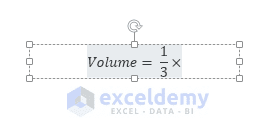I am inserting the formula…

Then there is a Pi sign in the equation, again Symbols, this time I select Greek Letters, from the lower case Greek letters, I select the Pi symbol. Then again multiplication sign.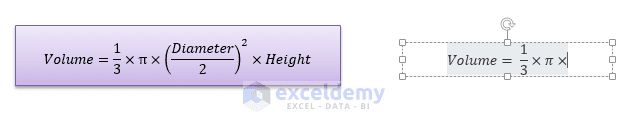The equation is not in this state…

In the equation now is: “diameter divided by 2” whole square. I select the Superscript structure, and I select the first blank box in the superscript, I insert parenthesis with single value from Bracket structure, I select the box within parenthesis, and I select the Stacked fraction structure again. At the upper box, I type Diameter, at the bottom I type just 2, and as the superscript, I type 2.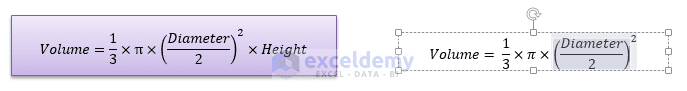The equation is now at this state.

Again press right arrow in the keyboard, the rest is simple, a cross sign and I type height. Our equation is complete, I format the shape of the equation editor, readjust the size, and align middle the equation in the shape and I click outside the equation editor. So we get the equation like the following image.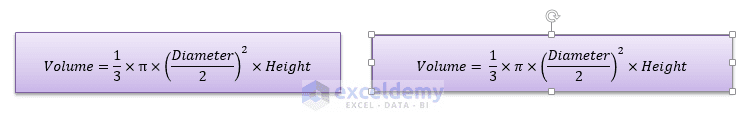Finally we get the equation.

### Inserting Second Equation

The second equation is simple. Let’s make it now. Before follow along me, try to make the equation by yourself at first.

Click on the Insert tab, Equation command, Equation Editor appears, type F, within parenthesis x, then equal sign, then from the Script structure select the Subscript, fill the blanks with “a” and “0”. Then insert a plus sign.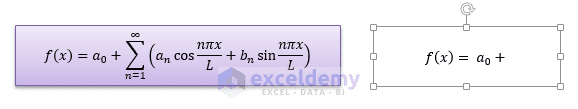Building the second equation…

Press right arrow in the keyboard, now in the equation, you will see a big sigma sign, in the Large Operator structure, select the second Summation structure, from the Basic Math window, find the infinity sign, then place “n equal to 1” in the lower part of the sigma.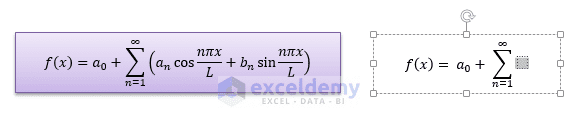Building the second equation…

Then I type a bracket. In the Bracket I type, a subscript n, then insert the cosine function, then a stacked fraction, at the top, I type n pi x, at the bottom I type L. Press right Arrow, place a plus sign,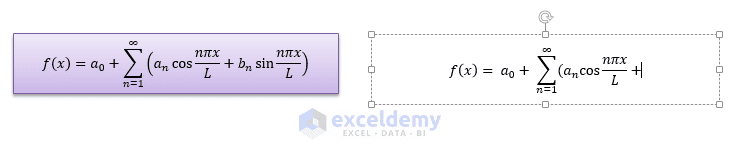Almost done with the second equation…

Then again the same structure.

Finally change the Shape format, and you are done.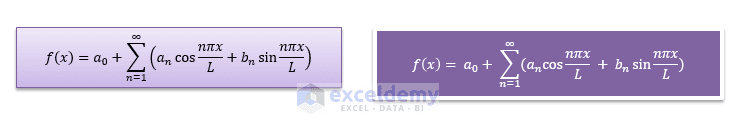Formatted equation.

So, I tried to explain you in step by step procedure how to use Excel equation editor to insert equations in Excel.

## Related Articles

Hello!
Welcome to my Excel blog! It took me some time to be a fan of Excel. But now I am a die-hard fan of MS Excel. I learn new ways of doing things with Excel and share here. Not only how to guide on Excel, but you will get also topics on Finance, Statistics, Data Analysis, and BI. Stay tuned!

1. ReplyJan 25, 2016 at 11:25 PM

Great tip Kawser!

In Excel 2016 this is even easier since you can use the Ink Equation feature to create equations manually.

This is explained in more detail here: https://support.office.com/en-us/article/What-s-new-in-Excel-2016-for-Windows-5fdb9208-ff33-45b6-9e08-1f5cdb3a6c73

Thanks,
Orlando

• ReplyKawser Jan 26, 2016 at 12:39 AM

Thanks Orlando for updating me 🙂
Regards
Kawser

2. ReplyBob Ritchie Jun 17, 2017 at 2:12 AM

I find the description totally incomprehensible. You instruct me to do something without explaining what I need to do and where to find it. You must assume I know nothing about this if teaching is you goal. It appears it is not. I have to be inside your head to follow.

• ReplyJun 17, 2017 at 3:49 PM

Hi Bob,
Did you download the Excel file before you follow me? Please do so. I’ve explained the whole process with two real time examples. If you can create these two examples in the Excel file, I hope you will be able to understand the whole system.
Best regards
Kawser

3. ReplyMike King Oct 24, 2017 at 8:51 PM

Thanks Kawser, I found it pretty easy to follow – I practiced on your download file. It sure seems pretty time consuming. But, it does make a fine looking formula!!

• ReplyOct 25, 2017 at 3:37 PM

Mike,
4. Reply